Question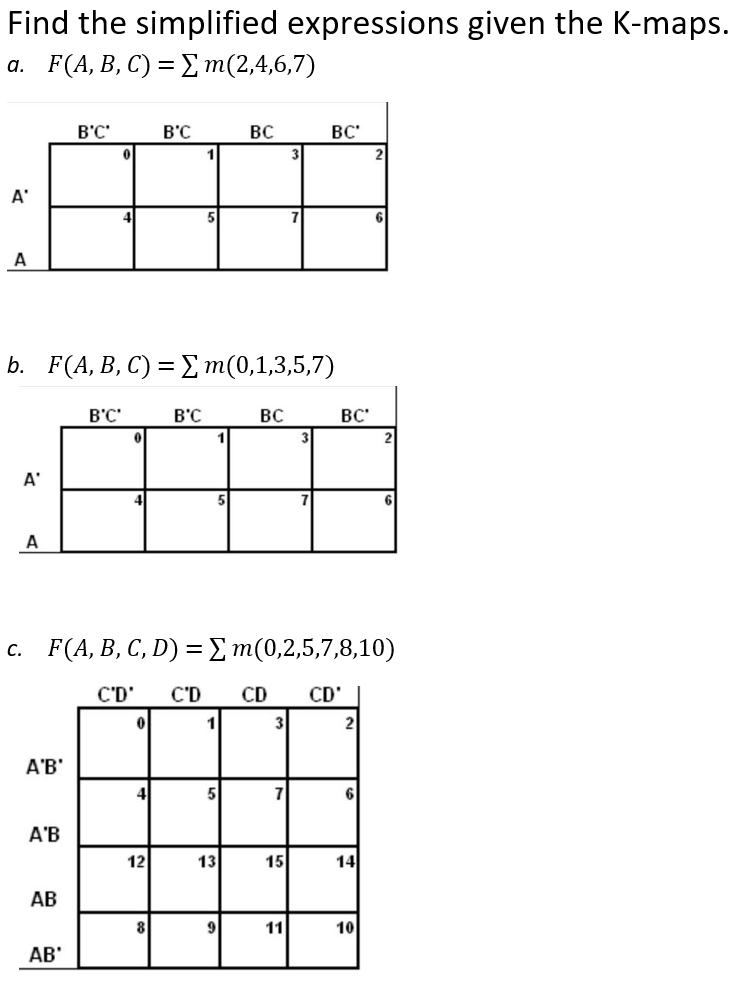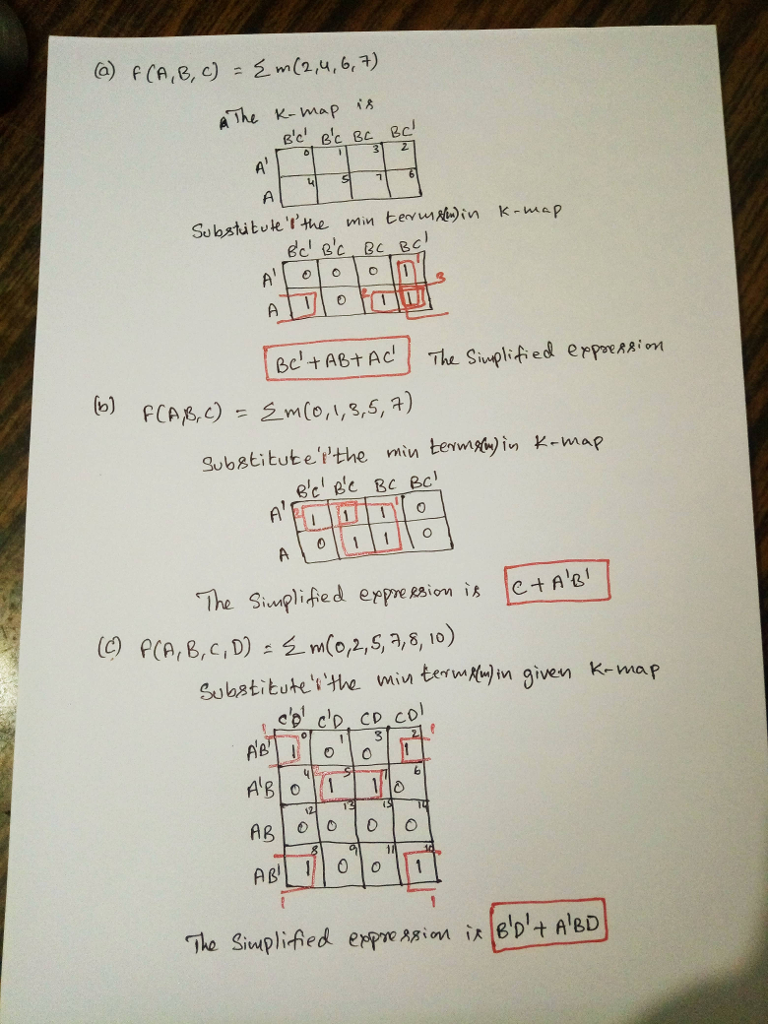#### Earn Coins

Coins can be redeemed for fabulous gifts.

Similar Homework Help Questions
• ### Simplify the following Boolean expressions, using four-variable maps. Draw a NAND only implementation of the simplified...

Simplify the following Boolean expressions, using four-variable maps. Draw a NAND only implementation of the simplified circuit. F(A,B,C,D) = A′B′C′D + AB′D + A′BC′ + ABCD + AB′C

• ### Using K-maps, obtain the simplified product-of-sums and sum-of-products expressions for the following Boolean functions: a). b)....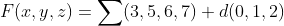Using K-maps, obtain the simplified product-of-sums and sum-of-products expressions for the following Boolean functions: a). b). F(x, y,2)-(3,5,6,7) d(0, 1,2) F(w,x, y, z) (0,1,2,3,7,8, 10)+ d(5,6,11, 15)

• ### draw k-maps and write the simplified equation for the following questions 1- f3(A, B, C, D)=m(1,...

draw k-maps and write the simplified equation for the following questions 1- f3(A, B, C, D)=m(1, 3, 4, 5, 6, 7, 9, 10, 11, 14, 15) 2- f4(A, B, C, D)=m(0, 1, 4, 6, 8, 9, 10, 12) + d(5, 7, 14) 3- g3(A, B, C, D)=M(0, 5,7, 14, 15) + d(3, 9, 13) 4- h(A, B, C, D, )= m(0, 1, 2, 4, 7, 8, 9, 10, 13, 14, 16, 17, 18, 19, 21, 23, 24, 25, 26,28, 30)...

• ### Use K-maps to find MSOP and MPOS expressions for the following incompletely specified functions. (a) f(a,b,c,d)...

Use K-maps to find MSOP and MPOS expressions for the following incompletely specified functions. (a) f(a,b,c,d) = ∑m(1,5,7,9) + d(6,13) (b) g(a,b,c,d) = ∏M(3,6,8,9,14) · D(1,2,4,7,11,12,13)

• ### Find the complement of the following expressions b) (AB+C)0%E 2. Given the Boolean function F -xy...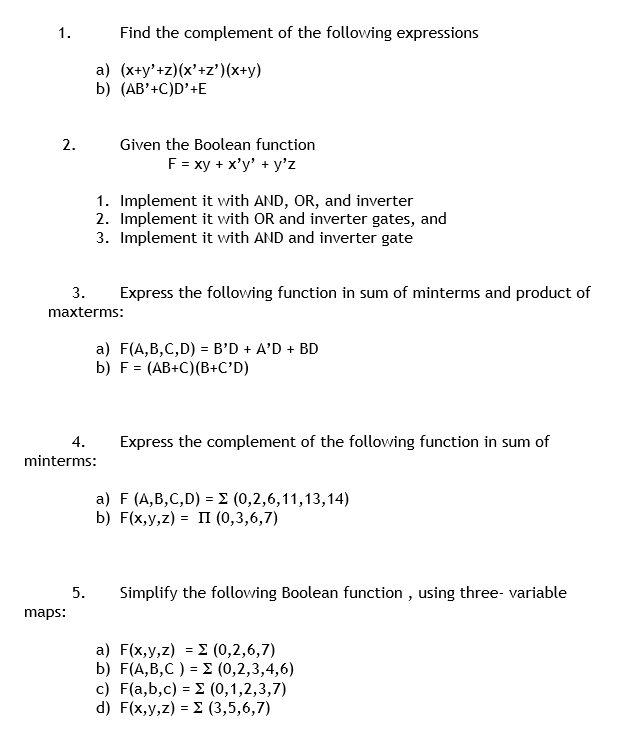Find the complement of the following expressions b) (AB+C)0%E 2. Given the Boolean function F -xy + x'y' y'z 1. Implement it with AND, OR, and inverter 2. Implement it with OR and inverter gates, and 3. Implement it with AND and inverter gate 3. Express the following function in sum of minterms and product of maxterms: a) F(A,B,C,D) - B'DA'D BD b) F (AB+C)(B+C'D) 4.Express the complement of the following function in sum of minterms a) F (A,B,C,D)-2 (0,2,6,11,13,14)...

• ### 1- DESIGN THE FOLLOWING CIRCUIT f(A,B,C,D) = Σ m( 0,1,3,4,6,8,9,11,13,15) in NAND implementation? 2- use a...

1- DESIGN THE FOLLOWING CIRCUIT f(A,B,C,D) = Σ m( 0,1,3,4,6,8,9,11,13,15) in NAND implementation? 2- use a K-map to minimize the following standard SOP expressions F(A,B,C)=A.C[B'+B.(B+C')]? 3- use a k-map to minimize the following standard POS expressions F(A,B,C)= A'.(BC+BC')+A,(BC+BC')

• ### 1. Use K-maps to reduce each of the following to a minimized SOP form: (a) A...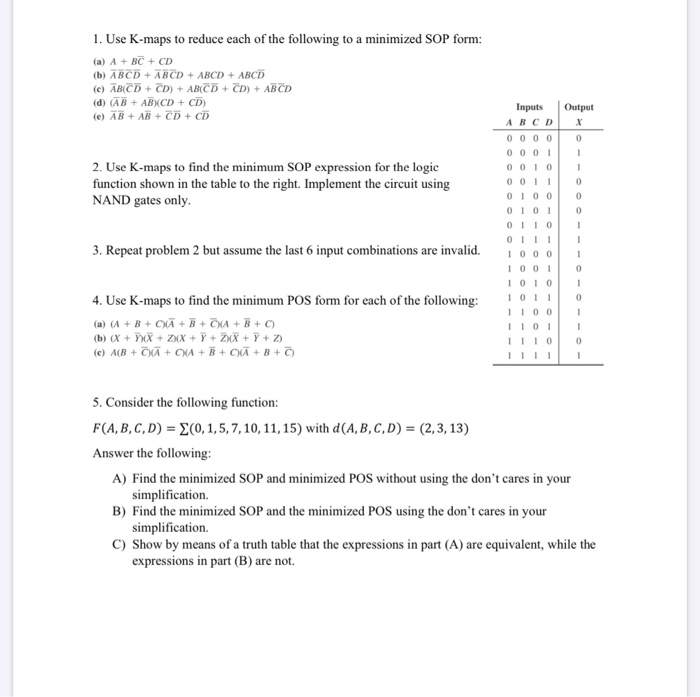1. Use K-maps to reduce each of the following to a minimized SOP form: (a) A + BC + CD (b) ABCD + ABCD + ABCD + ABCD (c) ABCD + CD) + ABCD + CD) + ABCD (d) (AB + ABXCD + CD) (e) AB + AB + CD + CD 2. Use K-maps to find the minimum SOP expression for the logic function shown in the table to the right. Implement the circuit using NAND gates only. Inputs...

• ### Given the semi-simplified Boolean equation below a) draw the K-map for F': b) find the simplified...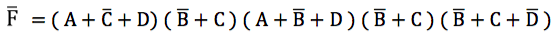Given the semi-simplified Boolean equation below a) draw the K-map for F': b) find the simplified SOP equation for F. F = (A + C + D) (B+C) (A+B+D (B+C)(B+C+ D)

• ### can help me to answer question b and c ,11, 12, 3.13 Simplify the following expressions...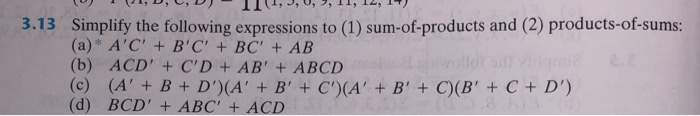can help me to answer question b and c ,11, 12, 3.13 Simplify the following expressions to (1) sum-of-products and (2) products-of-sums: (a) A'C' +B'C' +BC' + AB (b) ACD' + C'D + AB' + ABCD (c) ale (A' + B+ D')(A' + B' +C')( A' B' +C)(B' +C+ D') (d) BCD'+ABC' +ACD

• ### What is the simplified version of the four-variable K-map for the following expression ∗F(A,B,C,D)=Σ(0,2,4,5,6,7,8,10,13,15)

What is the simplified version of the four-variable K-map for the following expression ∗F(A,B,C,D)=Σ(0,2,4,5,6,7,8,10,13,15)